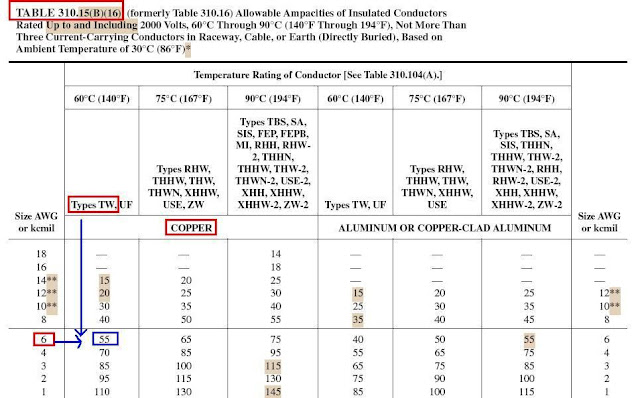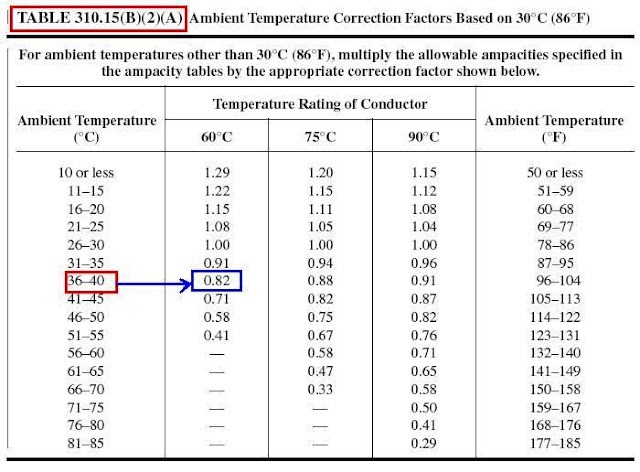# More Conductor Sizing and Overcurrent Protection Calculations

As I explained before in Article Conductor Ampacity Calculation – Part Three that:

 NEC Methods for Ampacity Calculations of Conductors Rated 0–2000 Volts As per 310.15(A)(1), The allowable Ampacities for conductors rated 0-2000 Volts shall be permitted to be determined by two methods: Tables as provided in 310.15(B) or Under engineering supervision, as provided in 310.15(C).

For more information about the first method for Conductors ampacity calculations by using Tables as provided in 310.15(B), please review the following articles:

For more information about the Second method for Conductors ampacity calculations by using Annex B Tables under engineering supervision, please review the following articles:

Today, I will explain the effect of Continuous vs. Non-continuous loads on conductor sizing and overcurrent protection.

Conductor Sizing and Overcurrent Protection Calculations for Continuous and Non-Continuous Loads

The type of the load (Continuous or Non-continuous) must be considered in The National Electrical Code requirements for conductor sizing and overcurrent protection.

 Important!!! The ampacities listed on the Tables in Article 310 are tested and approved for continuous use. However, the table ampacities can be used as continuous or non-continuous loads after all temperature and derating factors have been applied.

 Definitions Continuous loads: loads where the maximum current is expected to continue for 3 hours or more. It is for this reason that the conductors and their overcurrent protection devices face special rules to account for the increased heat that it will encounter during operation. Typical continuous loads are those such as lighting in an office, bank, etc. Outdoor signs are also assumed to be continuous when sizing conductors and over current protection.  Non-continuous loads: other loads than Continuous Loads.

 A- Sizing Overcurrent Protection 1- To find the maximum continuous load of a protection device, use the following formula: Maximum continuous load = OPD x .8 (or 80%) 2- To find the protection device of a continuous Load, use the following formula: Protection device size = 125% of continuous Load

Example#1:

What is the maximum continuous load placed on a 20 amp fuse or breaker?

Solution:

The maximum continuous load that can be applied to a 20 amp breaker = 20 A x .8 = 16 amps

Example#2:

What is the maximum continuous load of a 100 amp over current protection device?

Solution:

Maximum continuous load = 100 x .8 Maximum continuous load = 80 amps

Example#3:

What is the overcurrent protection device for an 80 amp continuous load?

Solution:

Using Articles 240.4(B) for “Protection of Conductors”, 240.6 for “Standard Ampere Ratings of overcurrent devices” and the formula for a continuous load:

OPD (continuous) = 80 amps x 1.25 OPD (continuous) = 100 amps

 B- Conductor sizing Calculations To Size The Conductors For Continuous Loads, Use The Following Steps: Step#1: Size the overcurrent protection device in accordance with Sections 210-20(a) for “Overcurrent Protection - Continuous and Noncontinuous Loads”. Step#2: Select the conductor to comply with Sections 110-14(c) for “Electrical Connections- Temperature Limitations” and 215.2 for “Feeders Minimum Rating and Size”. Section 215-2 also requires the feeder conductor to be sized no less than 125% of the continuous load. Step#3: The selected conductor must be protected against overcurrent in accordance with Section 240.4 for “Protection of Conductors”.

Example#4:

What size branch-circuit overcurrent protection device and conductor (THHN) is required for a 19 kVA of nonlinear loads (75°C terminals). The branch-circuit is supplied by a 208/120 volt, 4-wire, 3-phase, Wye connected system.

Solution:

Step#1: Size the overcurrent protection device in accordance with Sections 210-20(a) for “Overcurrent Protection - Continuous and Noncontinuous Loads”. The first thing that we must do convert the nonlinear load from kVA to amperes:

Amperes = VA/(Volts x 1.732), Amperes = 19,000/(208 volts x 1.732), Amperes = 52.74 amperes, rounded to 53 amperes

The branch-circuit overcurrent protection device must be sized not less than 125% of 53 amperes,

So, branch-circuit overcurrent protection device size = 53 amperes x 125% = 66 amperes.

According to Section 240.6(a) for “Standard Ampere Ratings of overcurrent devices”, we must select a minimum 70 ampere overcurrent protection device.

Step#2: Select the conductor to comply with Sections 110-14(c) for “Electrical Connections- Temperature Limitations” and 210-19(a) for “Conductors — Minimum Ampacity and Size - Branch Circuits Not More Than 600 Volts”. Section 210-19(a) also requires the branch-circuit conductor to be sized no less than 125% of the continuous load,

So, branch-circuit conductor amps = 53 amperes x 125% = 66 amperes.

We must select the conductor according to the 75°C terminals temperature rating of the equipment terminals. No. 6 THHN has a rating of 65 amperes at 75°C and cannot be used, therefore we must select a No. 4 which has a rating of 85 amperes at 75°C.

Step#3: The No. 4 THHN conductor must be protected against overcurrent in accordance with Section 240.4 for “Protection of Conductors”. We must verify that the No. 4 THHN is properly protected against overcurrent by the 70 ampere overcurrent protection device.

Since we have more than three current-carrying conductors in the same raceway, we must correct the No. 4 THHN conductors ampacity as per table 310.15(B)(3)(A), Derating Factor = 0.80 for THHN

So, Corrected Ampacity No. 4 THHN = Ampacity x
Derating  Factors from table 310.15(B)(3)(A)

Corrected Ampacity No. 4 THHN = 95 amperes x 0.80 = 76 amperes

The No. 4 THHN which is rated 76 amperes after ampacity correction is properly protected by a 70 ampere overcurrent protection device in compliance with the general requirements of Section 240.4 for “Protection of Conductors”.

Example#5:

What size feeder overcurrent protection device and conductor (THHN) is required for a 184 ampere continuous load on a panelboard (75°C terminals) that supplies nonlinear loads. The feeder is supplied by a 4-wire, 3-phase, wye connected system.

Solution:

Step#1: Size the overcurrent protection device in accordance with Sections 215-3 for “feeders Overcurrent Protection” . The feeder overcurrent protection device must be sized not less than 125% of 184 amperes,

So, overcurrent protection device size = 184 amperes x 125% = 230 amperes.

According to Section 240-6(a) for “Standard Ampere Ratings of overcurrent devices”, we must select a minimum 250 ampere overcurrent protection device.

Step#2: Select the conductor to comply with Sections 110-14(c) for “Electrical Connections- Temperature Limitations”and 215-2. Section 215-2 for “Feeders Minimum Rating and Size”also requires the feeder conductor to be sized no less than 125% of the continuous load,

Feeder conductor amps = 184 amperes x 125% = 230 amperes.

We must select the conductor according to the 75°C temperature rating of the panelboards terminals. No. 4/0 THHN has a rating of 230 amperes at 75°C.

Step#3: The No. 4/0 conductor must be protected against overcurrent in accordance with Section 240-4 for “Protection of Conductors”. We must verify that the No. 4/0 THHN conductor is properly protected against overcurrent by the 250 ampere overcurrent protection device. Since we have more than three current-carrying conductors in the same raceway, we must correct the No. 4/0 THHN conductors ampacity as per table 310.15(B)(3)(A),
Derating Factor = 0.80 for THHNSo, Corrected Ampacity No. 4/0 THHN = Ampacity x
Derating  Factors from table 310.15(B)(3)(A)

Corrected Ampacity No. 4/0 THHN = 260 amperes x 0.80 = 208 amperes

The No. 4/0 THHN which is rated 208 amperes after ampacity correction is not considered protected by a 250 ampere overcurrent protection device. This is because "the next size up rule" in Section 240-4(b) would only permit a 225 ampere protection device on the 208 ampere conductor [240-6(a)]. Therefor we must increase the conductor size to 250 kcmil in order to comply with the overcurrent protection rules of Section 240-4 for “Protection of Conductors”.

 A- Sizing Overcurrent Protection  1- To find the maximum non-continuous load of a protection device, use the following formula:  Maximum non-continuous load = 100% of OPD Rating  2- To find the protection device size of a non-continuous Load, use the following formula:  Protection device size = 100% of non-continuous Load  Note: no special consideration or increased loading factors are given to non-continuous loads.

 B- Conductor sizing Calculations  To Size the Conductors For non-Continuous Loads, Use the Following Steps:  Step#1: Size the overcurrent protection device in accordance with Sections 240.6 for "Standard Ampere Ratings of overcurrent protective devices" and 240.4(B) for "Protection of Conductors".  Step#2: If ambient temperature and/or more than three conductors are involved, then calculate Wire ampacity needed from table from the following formula: Wire ampacity needed from table = load at 100% (Non-Continuous) / (Temp Correction Factors from table 310.15(B)(2)(A) x derating factor from table 310.15(b)(3)(a))  Step#3: Select the conductor from Article 310 tables based on the calculated ampacity from step#2.

Example#6:

What size circuit breaker and THW conductor would you need for non-continuous load of 73 amps?

Solution:

Step#1: Protection device size = 100% of non continuous load
So, Protective device 80 amps, Article 240.4(B), 240.6

Step#2: no ambient temperature and no more than three conductors are involved
So, step#2 is not applicable

Step#3: Using Table 310.15(B)(16), we find the wire size needed to be #4THW copperExample#7:

There is a 34 amp load and the raceway is installed over a roof where the ambient temperature is 110° F, 2 wire circuit. Assume a non-continuous load.

What size protection device is required?
What size RHW conductor is required?

Solution:

Step#1: Protection device size = 100% of non continuous load
So, Protection device size = 35 amps, 240.4(B), 240.6

Step#2: Wire ampacity needed from table = load at 100% (Non-Continuous) / (Temp Correction Factors from table 310.15(B)(2)(A) x derating factor from table 310.15(b)(3)(a))

Load at 100% (Non-Continuous) = 34 ampsAmbient Temperature = 110°F, so from table 310.15(B)(2)(A), Temp Correction Factors = 0.82 for RHW

Because there are only 2 wires, no derating factor from table 310.15(b)(3)(a) will be applied

So, Wire ampacity needed from table = 34 amps / 0.82 = 41.46 amps

Step#3: Using Table 310.15(B)(16), we find the wire size needed to be #8 RHWExample#8:

There are ten current carrying conductors installed in an ambient temperature of 57°C. A 48 amp load 2-wire circuit must be added.

What size protection device is required for the 48 amp load?
What size THHN copper conductor is required for the 48 amp load?

Solution:

Step#1: Protection device size = 100% of non-continuous load.
From 240.4(B), 240.6, Protection device size = 50 amps

Step#2: Wire ampacity needed from table = load at 100% (Non-Continuous) / (Temp Correction Factors from table 310.15(B)(2)(A) x derating factor from table 310.15(b)(3)(a))
Load at 100% (Non-Continuous) = 48 amps

Ambient Temperature = 57°C, so from table 310.15(B)(2)(A), Temp Correction Factors = 0.71 for THHN

(10) nos. existing current carrying conductors plus 2 new current carrying conductors = 12 current carrying conductors. Using 310.15(b)(3)(a) gives us a derating factor of 50%

So, Wire ampacity needed = 48 amps / (0.71 x 0.5) = 135.3 amps

Step#3: Using Table 310.15(B)(16), we find the wire size needed to be #1 THHN copperExample#9:

We have four current carrying conductors in a raceway and two are #6TW. The ambient temperature is 40° C.

What is the new ampacity?
What size is the protection device?

Solution:

First: New Ampacity = Conductor Ampacity x Temp Correction Factors from table 310.15(B)(2)(A) x derating factor from table 310.15(b)(3)(a)Conductor Ampacity from Table 310.15(B)(16) for #6 TW = 55 AAmbient Temperature = 40°C, so from table 310.15(B)(2)(A), Temp Correction Factors = 0.82 TW

(4) nos. current carrying conductors, so by Using 310.15(b)(3)(a) gives us a derating factor of 80%

New Ampacity = 55 amps x 0.82 x 0.8 = 36.1 amps

Second: Protection device size = 100% of non-continuous load.

From 240.4(B), 240.6, Protection device size = 40 amps

In the next Article, I will explain the NEC Grounding Rules and Calculations. Please, keep following.